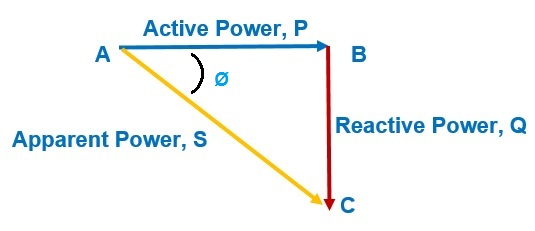# Poor Power Factor – Effects and Disadvantage

### Effect of Poor Power Factor:

The major effect of poor power factor is higher value of line current. We know that power factor (pf) is an important parameter for calculation of power in an AC circuit. For a given power and voltage, the current flowing through the line is inversely proportional to the power factor. This means that a poor power factor i.e. low power factor will result in higher load current and hence higher losses.

Thus it is always desirable to achieve unity power factor. But it is not possible to design equipment having pf unity. But efforts are made to achieve unity power factor by power factor improvement using correction devices like capacitor.

Power calculation for single phase system and three phase system are given as

P = VLILCosØ

Where VL = Line voltage

IL = Line current and CosØ = pf

Thus current IL = P / VLCosØ

Similarly, for three phase system

Power P = √3VLILCosØ

IL = P / √3VLCosØ

From the above expressions of current in single phase as well as three phase system, we observe that current is inversely proportional to CosØ i.e. power factor as stated earlier.

### Disadvantage of Poor Power Factor:

Following are the effects of poor power factor due to which its value should be improved or corrected:

• Requirement of large kVA Rating of Equipment:

Electrical equipment / machines are generally rated in kVA. But as per the power triangle∴ CosØ = P / S = kW / kVA

⇒ kVA = kW / CosØ

Thus, lower value of CosØ will lead to higher value of kVA rating. This in turn will result in higher machine losses. Therefore the size of machine will increase and hence its cost will also increase.

• Greater Conductor Size:

Poor power factor is not only important for loads but it also plays an important role is power transmission. As discussed, low power factor causes higher line current. As the current carrying capacity of a conductor is directly proportional to its cross-sectional area, higher current will require greater conductor size. Thus the size of transmission conductor increase.

• Large Copper Losses:

Ohmic loss (I2r loss) is also known as copper loss. Larger current due at low power factor causes more ohmic loss and hence reduced efficiency.

• Poor Voltage Regulation:

Percentage Voltage Regulation of Transmission Line is given as

% Voltage Regulation = [(VS – VR) x100/ VR ] %

Since large line current causes larger voltage drop in the line impedance. Therefore the receiving end voltage (VR) will be much lower than the sending end voltage (VS). Hence voltage regulation will be poor.

### Why Power Factor Improvement Required?

Keeping all the disadvantages of poor power factor in mind, we can realize the necessity of power factor correction or improvement. Power factor improvement not only eliminates the problems associated with low power factor but it also makes the system economic. Power factor can be improved by application of either of capacitor, synchronous condenser or phase advancer.

Suppose an industry have existing pf value of 0.6. An improvement in its value to 0.95 is desired. Then either we may connect a capacitor or synchronous condenser in parallel with the load. The value of the correction capacitor or synchronous condenser may be calculated by power factor correction formula. Power Factor Calculator is made using this correction formula. You just need to download this calculator and input some variables. You will get the value of required correction capacitor and synchronous condenser. You can download this Calculator from the link below. Don’t forget to comment about this calculator.

### 4 thoughts on “Poor Power Factor – Effects and Disadvantage”

1.M.Raman says:

Wow! This is excellent Sir! This power factor calculator is just awesome.. It gives the precised value of power factor. There are so many other options too!
Thank You so much!

1.admin says:

Thank you M. Raman!

2.Takunda Vito says:

I appreciate the explanation it is short precise and clear

1.admin says:

Thank you. Request you to kindly share.

This site uses Akismet to reduce spam. Learn how your comment data is processed.